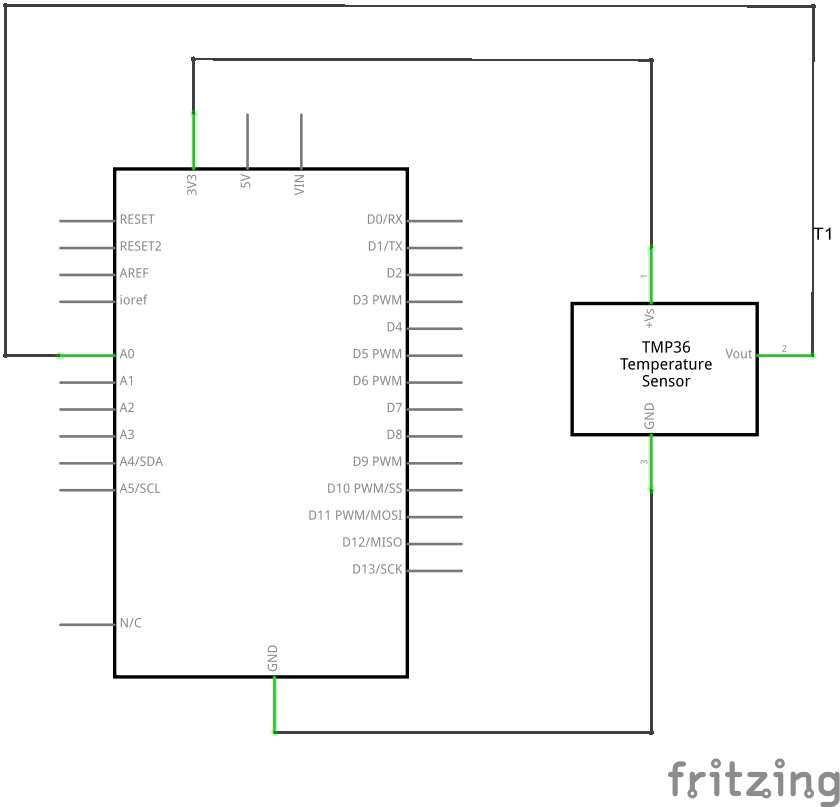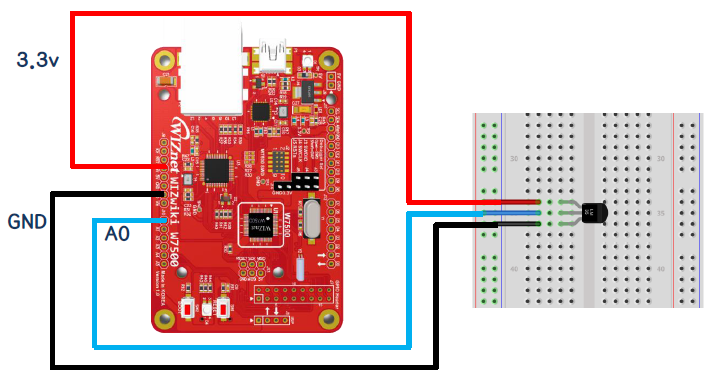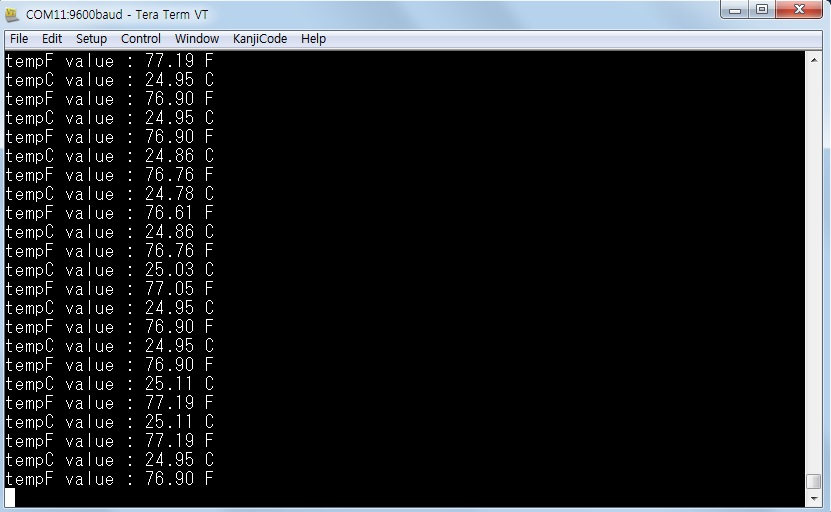Skip to main content

# Reading the temperature sensor value

## Summary#

This is an example of reading analog temperature sensor value using ADC. Convert analog values ​​to Celsius and Fahrenheit using formulas. The converted value It is displayed on the screen through the serial port..

## Materials#

• WIZwiki-W7500 board
• USB cable
• Breadboard
• Jumper wire
• Temperature Sensor TMP36GT9 (Low Voltage Temperature Sensor)

## Hardware#

### Schematic#

The overall circuit diagram is as follows.### Connection diagram### Software#

### Example Code#

Here is an example code.

Open the page below and compile by importing the program with "Import this program".

Or, compile after copying and pasting the following code to main.c in the mbed environment.

``/* Analog Input "Temp36" Temperature Sensor Example Program */ #include "mbed.h" // Initialize a pins to perform analog input fucntionsAnalogIn   ain(A2); // connect A0(WIZwiki-W7500) to Vout(Temp36) int main(void){    while (1)    {        float V = ain.read(/) * 3.3; // connect Vs(Temp36) to 3.3V(WIZwiki-W7500)         //float V = ain.read(/) * 5; // connect Vs(Temp36) to 5V(WIZwiki-W7500)                float tempC = (V-0.5) * 100; // calculate temperature C        float tempF = (tempC * 9 / 5) + 32.0; // calculate temperature F                printf("tempC value : %5.2f C \r\n", tempC);        printf("tempF value : %5.2f F \r\n", tempF);                wait(1.0);    }}``

### Methods and results#

The following is an example execution screen. Celsius and Fahrenheit temperature values alternate.## Learning materials#

See the link below for temperature sensor data.

• [Kit Configuration Data Sheet] (/)